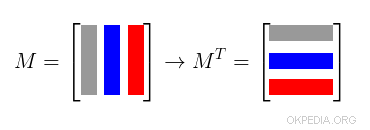# How to calculate the transpose of a matrix in Matlab and Octave

To calculate the transpose of a matrix in Matlab or Octave use the function transpose()

transpose(m)

Alternatively, write the array in this syntax

m'

or in this syntax

m.'

The parameter m is the matrix.

In both cases the transposed matrix of m is generated.

What is a transposed matrix? The transpose MT of a matrix M is the matrix in which the columns of the matrix M are transformed into rows (or vice versa).## Examples

Example 1

Create a matrix 3x3 matrix

>> A = [1,2,3;4,5,6;7,8,9]
1 2 3
4 5 6
7 8 9

The transpose() function calculates the transpose of matrix A

>> transpose(A)
1 4 7
2 5 8
3 6 9

Example 2

The same result is obtained by writing A'

>> A'

The result is the transposed matrix of A

1 4 7
2 5 8
3 6 9

https://how.okpedia.org/en/matlab/how-to-calculate-the-transpose-of-a-matrix-in-matlab-and-octaveReport us an error or send a suggestion to improve this page

Matrix in Matlab/Octave